# Top Machine Learning Interview Questions for 2019 (Part-1)These Machine Learning Interview Questions, are the real questions that are asked in the top interviews.

For hiring machine learning engineers or data scientists, the typical process has multiple rounds.

1. A basic screening round – The objective is to check the minimum fitness in this round.
2. Algorithm Design Round – Some companies have this round but most don’t. This involves checking the coding / algorithmic skills of the interviewee.
3. ML Case Study – In this round, you are given a case study problem of machine learning on the lines of Kaggle. You have to solve it in an hour.
4. Bar Raiser / Hiring Manager  – This interview is generally with the most senior person in the team or a very senior person from another team (at Amazon it is called Bar raiser round) who will check if the candidate fits in the company-wide technical capabilities. This is generally the last round.

A typical first round of interview consists of three parts. First, a brief intro about yourself.

Second, a brief about your relevant projects.

A typical interviewer will start by asking about the relevant work from your profile. On your past experience of machine learning project, the interviewer might ask how would you improve it.

Say, you have done a project on recommendation, the interviewer might ask:

• How would you improve the recommendations?
• How would you do ranking?
• Have you done any end-to-end machine learning project? If yes, then what were the challenges faced. How would you do solve the problem of the cold start?
• How would you improve upon the speed of recommendation?

Afterwards (third part), the interviewer would proceed to check your basic knowledge of machine learning on the following lines.

## Q1: What is machine learning?

Machine learning is the field of study that gives the computer the ability to learn and improve from experience without explicitly taught or programmed.

In traditional programs, the rules are coded for a program to make decisions, but in machine learning, the program learns based on the data to make decisions.

## Q2. Why do we need machine learning?

The most intuitive and prominent example is self-driving cars, but let’s answer this question in the more structured way. Machine learning is needed to solve the problems that are categorized as below:

• Problems for which a traditional solution require a long and complex set of rules and requires hand-tuning often. Example of such problem is email spam filter. You notice a few words such as 4U, promotion, credit card, free, amazing etc. and figure out that the email is a spam. This list can be really long and can change once the spammer notices that you started ignoring these words. It becomes hard to deal with this problem with traditional programming approach. Machine learning algorithm learns to detect spam emails very well and works better.
• Complex problems for which there is no good solution at all using the traditional approach. Speech recognition is an example of this category of the problems.
Machine learning algorithms can find a good solution to these problems.
• Fluctuating environment: a machine learning system can adapt to new data and learn to do well in this new set of data.
• Getting insights into complex, large amounts of data. For example, your business collects a large amount of data from the customers. A machine learning algorithm can find insights into this data which otherwise is not easy to figure out.

For more details visit Machine Learning Specialization

## Q3. What is the difference between the supervised and unsupervised learning? Give examples of both.

By definition, the supervised and unsupervised learning algorithms are categorized based on the supervision required while training. Supervised learning algorithms work on data which are labelled i.e. the data has a desired solution or a label.

On the other hand, unsupervised learning algorithms work on unlabeled data, meaning that the data does not contain the desired solution for the algorithm to learn from.

Supervised algorithm examples:

• Linear Regression
• Neural Networks/Deep Learning
• Decision Trees
• Support Vector Machine (SVM)
• K-Nearest neighbours

Unsupervised algorithm examples:

• Clustering Algorithms – K-means, Hierarchical Clustering Analysis (HCA)
• Visualization and Dimensionality reduction – Principal component reduction (PCA)
• Association rule learning

## Q4. Is recommendation supervised or unsupervised learning?

Recommendation algorithms are interesting as some of these are supervised and some are unsupervised. The recommendations based on your profile, previous purchases, page views fall under supervised learning. But there are recommendations based on hot selling products, country/location-based recommendations, which are unsupervised learning.

For more details visit Machine Learning Specialization

## Q5. Explain PCA?

PCA stands for Principal Component Analysis. PCA is a procedure to reduce the dimensionality of the data, which consist of many variables related to each other heavily or lightly while retaining the variation in the data to the maximum possible. The data on which the PCA is applied has to be scaled data and the result of the PCA is sensitive to the relative scaling of the data.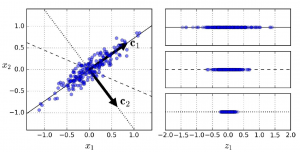PCA – Hyperplanes

For an example, say, you have dataset in 2D space and you would need to choose a hyperplane to project the dataset. The hyperplane must be chosen such that the variance is preserved to the maximum. In below figure, when converting from one representation to another (left to right), the hyperplace C1 (solid line) has preserved maximum variance in the dataset while C2 (dotted line) has very little variance preserved.

## Q6. Which supervised learning algorithms do you know?

You need to answer this question in your own comfort level with the algorithm. There are many supervised learning algorithms such as regression, decision tree, neural networks, SVM etc. Out of these the most popular and simple algorithm in supervised learning is the linear regression. Let me explain it in a quick way.

Say we need to predict income of residents of a county based on some historical data. Linear regression is can be used for this problem.
The linear regression model is a linear function of input features with weights which define the model and a bias term as shown below.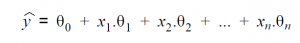In this equation y_hat  is the predicted outcome, x_are the inputs and theta_i are the model parameters or weights. theta_is the bias.
The performance of this model is measured by evaluating Root Mean Square Error (RMSE). In practice, Mean Square Error is minimized to find the values so that the MSE is the least.MSE is given as below: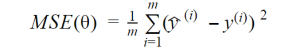## Q7. Can you compare Decision Trees and linear regression? Can decision trees be used for non-linear classification?

Decision trees are used for both unsupervised and supervised learning. Also, they are used for classification as well as the regression in supervised machine learning problems. In decision trees, we form the tree by splitting the node. Initially, all of the instances are divided into two parts based on a boundary such that the instance on either side is boundary is very close to other instance on the same side. The instances on the left-hand side should be very similar to other instance on the left-hand side and same is true for the right-hand side.

Below figure shows the decision tree of max depth 2 and max depth 3; you can see that as the max depth of the decision tree increases you get a better coverage of the available data.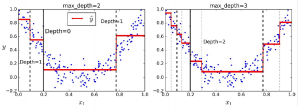Decision Trees with Different Depths

One more aspect of the decision tree worth highlight is the stability of the decision trees. The decision trees are sensitive to the dataset rotations. Below picture demonstrates the instability of decision tree while the data is rotated.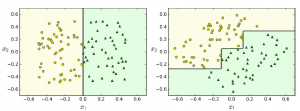Decision Tree Sensitivity with Rotation of Data

For more details visit Machine Learning Specialization

## Q8. Explain overfitting and underfitting? What causes overfitting?

Say, there are two kids Jack and Jill in a maths exam. Jack only learnt additions and Jill memorized the questions and their answers from the maths book.  Now, who will succeed in the exam? The answer is neither. From machine learning lingo, Jack is underfitting and Jill is overfitting.

Overfitting is failing of the algorithm to generalize to new examples which are not in the training set, at the same time the algorithm works very well for training set data same as Jill can answer the question which is in the book but nothing besides it. Underfitting, on the other hand, refers to the model when it does not capture the underlying trend of the data (training data as well as test data). The remedy, in general, is to choose a better (more complex) machine learning algorithm.

So, the underfitting models are the ones that give bad performance both in training and test data. Overfitting is very important to keep a tab on while developing the machine learning algorithms. This is because, by intuition, if the model fits very well with the training set the developers tend to think that the algorithm is working well, sometimes failing to account for overfitting. Overfitting occurs when the model is too complex relative to the amount and noisiness of the training data. It also means that the algorithm is not be working for test data well, maybe because the test data does not come from the same distribution as that of training data. Below are some of the ways to avoid overfitting:

• Simplify the model: regularization, controlled by hyperparameter
• Gather more training data
• Reduce the noise in the training data

Below are some of the ways to avoid underfitting:

• Selecting a more powerful model
• Feeding better features to the learning algorithm
• Reducing the constraints on the model (reduce regularization hyperparameter)

## Q9. What is cross-validation technique?

Let’s understand what validation set is and then we will go to cross-validation. When building the model, the training set is required to tune the weights by the means of backpropagation. And these weights are chosen such that the training error is minimum.

Now you need data to evaluate the model and the hyperparameters and this data can not be the same as the training set data. Hence a portion of the training set data is reserved for validation and is called the validation set. When testing different models to avoid wasting too much data in the validation of the models by keeping separate validation sets, the cross-validation technique is used. In cross-validation technique training data is divided into complimentary sub-sets and a different set of training and validation set are used for different models.

Then finally the best model is tested with test data.

For more details visit Machine Learning Specialization

## Q10. How would you detect overfitting and underfitting?

This is one of the most important questions of practical machine learning. For answering this question, let’s understand the concept of bias and variance.

In order to conclude whether the algorithm is overfitting or underfitting, you need to find out the training set error (E_train) and cross-validation set error (E_cv). If your E_train is high and E_cv is also in the same range as E_train i.e. both E_train and E_cv are high. This is the case of high bias and the algorithm is underfitting. In another case, say, your training set error is low but your cross-validation set error is high: E_train is low and E_cv is high. This is the case of high variance and the algorithm is overfitting.

## Q11. What’s the trade-off between bias and variance?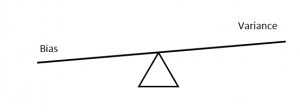Bias vs Variance

In simple terms, you can understand that a very simple algorithm (which does not capture the underlying details of the data) underfit, and has high bias and a very complex algorithm overfit and has high variance. There has to be a balance between the two. The picture below depicts how they are related in terms of the trade-off between them.

## Q12. How would you overcome overfitting in the algorithms that you mentioned above?

As mentioned above, the ways to overcome overfitting are as below:

• Simplify the model: regularization, controlled by hyperparameter
• Gather more training data
• Reduce the noise in the training data

## Q13. There is a colleague who claims to have achieved 99.99% accuracy in the classifier that he has built? Would you believe him? If not, what could be the prime suspects? How would you solve it?

99.99% is a very high accuracy, in general, and should be suspected. At least a careful analysis of the data set and any flow in modelling the solution around it be checked thoroughly. My prime suspects would be the data set and the problem statement. For example: in a set of handwritten characters where there are digits from 0 to 9 and if one builds a model to detect whether a digit is 5 or not 5. A faulty model which always recognize a digit as 8 will also give 90% accuracy, given all digits have the equal number of images in the data set.
In this case, the data set is not having good distribution for the problem of detecting 5 or not 5.

## Q14. Explain how a ROC curve works?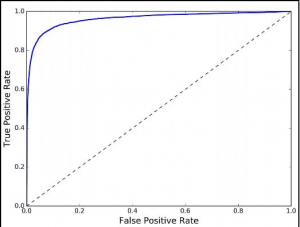ROC Curve

ROC stands for Receiver Operating Characteristic. ROC curve is used to measure the performance of different algorithms. This is a measurement of the area under the curve when the true positive rate and the false positive rate is plotted. More the area better the model.

For more details visit Machine Learning Specialization

## Q15. Explain the ensemble methods? What is the basic principle?

Say you ask a question to thousands of people and then aggregates the answer, many times this answer is better than an expert’s answer. Ensemble methods are basically combining the predictions of different learning algorithms such as classification, regression etc., to achieve a higher accuracy. This aggregate prediction is better than the best individual predictor. These group of predictors are called ensemble and the technique is called ensemble learning.

## Q16. Say, you have a dataset having city id as the feature, what would you do?

When you collect the data for your machine learning project, you need to carefully select the features from the data collected. City id is just a serial number which does not represent any property of the city unless otherwise stated, so I would just drop city id from the features list.

## Q17. In a dataset, there is a feature hour_of_the_day which goes from 0 to 23. Do you think it is okay?

This feature can not be used as it is because of the simple reason that hour_of_the_day may imply a certain constraint on your problem to be solved using the machine learning technique but there is a flaw to use the feature as is. Consider 0 and 23, these two numbers have a large numeric difference but in fact, they are close in the actual occurrence in the day, hence the algorithm may not produce desired results. There are two ways to solve this. First is to apply sine function with the periodicity of 24 (hours in a day), this will result in a continuous data from a discontinuous data.

The second approach is to divide the hours of the day into categories such as morning, afternoon, evening, night etc. or in the split of peak hours and non-peak hours based on your knowledge of the domain of your problem.

## Q18. If you have a smaller dataset, how would handle?

There are multiple ways to deal with this problem. Below are a few techniques.

1. Data augmentation
2. Pretrained Models
3. Better algorithm
4. Get started with generating the data
5. Download from internet

To learn more in details, join the course on machine learning## Author: Devilal Sharma

Devilal graduated from IIT Madras in 2006 with B. Tech and M. Tech (Dual Degree) in Electrical Engineering with specialization in Communication Systems. He has 12 years of experience in Software Development in various layers of software stack. Currently he is involved in solving challenging machine learning problems from our industry partners. He is actively involved at CloudxLab. Having completed multiple machine learning and related certification courses from Coursera/Udacity, he feels CloudxLab is a next level, disruptive platform. He commands machine learning areas and software engineering with skills such as Java, Python, NumPy, Spark, Tensorflow, Keras, OpenCV, Matlab, C and web technologies. In past, he has also worked extensively on multimedia (audio, speech, video) algorithms, codecs and frameworks (e.g. android multimedia framework) for mobile SOCs and IoT products. You can send him a note for any query.